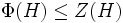# Analogue of critical subgroup theorem for infinite abelian-by-nilpotent p-groups

## Statement

Suppose$p$ is a prime number and$G$ is an infinite P-group (?) that is Abelian-by-nilpotent group (?):$G$ has an abelian normal subgroup$N$ such that the quotient$G/N$ is a nilpotent group. Then,$G$ has a characteristic subgroup$H$ satisfying the following properties:

1.$\Phi(H) \le Z(H)$, viz., the Frattini subgroup is contained inside the center (i.e.,$H$ is a Frattini-in-center group (?)).
2.$[G,H] \le Z(H)$ (i.e.,$H$ is a Commutator-in-center subgroup (?) of$G$).
3.$C_G(H)= Z(H)$ (i.e.,$H$ is a Self-centralizing subgroup (?) of$G$).
4.$H$ is coprime automorphism-faithful in$G$: If$\sigma$ is a non-identity automorphism of$G$ such that the order of$\sigma$ is relatively prime to$p$, then the restriction of$\sigma$ to$H$ is a non-identity automorphism of$H$.

This a the generalization of a critical subgroup to a possibly infinite p-group.

## Facts used

1. Equivalence of definitions of abelian-by-nilpotent group: This states that$G$ is abelian-by-nilpotent if and only if some member of its lower central series is abelian; in particular, that member of the lower central series is an abelian characteristic subgroup with a nilpotent quotient group.
2. Every abelian characteristic subgroup is contained in a maximal among abelian characteristic subgroups
3. Characteristic implies normal
4. Third isomorphism theorem
5. Nilpotence is quotient-closed

## Proof

The proof of the result is exactly the same as for finite groups. The main tricky first step is to show that there exists a subgroup that is Maximal among abelian characteristic subgroups (?), and such that the quotient is a nilpotent group. We do this first step in a separate subsection.

### There exists a subgroup maximal among abelian characteristic subgroups with a nilpotent quotient group

Given: A group$G$ with an abelian normal subgroup$N$ such that$G/N$ is nilpotent.

To prove: There exists a subgroup$K$ of$G$ such that$G/K$ is nilpotent and$K$ is maximal among abelian characteristic subgroups.

Proof:

1. There exists an abelian characteristic subgroup$L$ of$G$ such that$G/L$ is nilpotent: This is a consequence of fact (1).
2.$L$ is contained in a subgroup$K$ that is maximal among abelian characteristic subgroups: This follows from fact (2).
3.$K$ is abelian characteristic and$G/K$ is abelian: By fact (3), both$K$ and$L$ are normal in$G$, and by fact (4), we have$(G/L)/(K/L) \cong G/K$. Thus,$G/K$ is isomorphic to a quotient of a nilpotent group. By fact (5),$G/K$ is nilpotent.